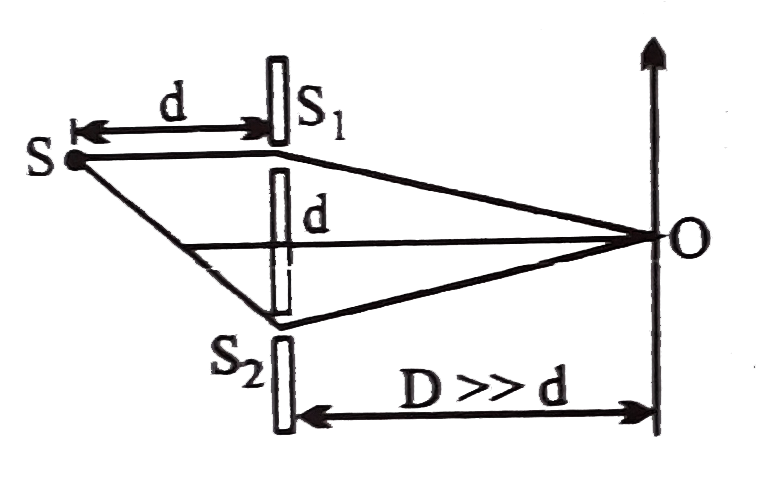# To make the central fringe at the centerO , mica sheet of refractive index 1.5 is introduced Choose the corect statement.

19 views
in Physics
To make the central fringe at the centerO , mica sheet of refractive index 1.5 is introduced Choose the corect statement.A. The thickness of sheet is (d^(2))/(D) in front of S_(1)
B. The thickness of sheet is (d^(2))/(D) in front of S_(2)
C. The thickness of sheet is (d^(2))/(2D) in front of S_(1)
D. The thickness of sheet is (d^(2))/(2D) in front of S_(1)

by (73.0k points)